# The inverse problem of the heat equation with periodic boundary and integral overdetermination conditions

## Abstract

In this paper the inverse problem of finding the time-dependent coefficient of heat capacity together with the solution of a heat equation with periodic boundary and integral overdetermination conditions is considered. The conditions for the existence and uniqueness of a classical solution of the problem under consideration are established. A numerical example using the Crank-Nicolson finite-difference scheme combined with the iteration method is presented.

## 1 Introduction

In this paper we consider an inverse problem of simultaneously finding the unknown coefficient $p\left(t\right)$ and the temperature distribution $u\left(x,t\right)$ that satisfy the equation

${u}_{t}={u}_{xx}-p\left(t\right)u+F\left(x,t\right),\phantom{\rule{1em}{0ex}}0
(1)

with the initial condition

$u\left(x,0\right)=\phi \left(x\right),\phantom{\rule{1em}{0ex}}0\le x\le 1,$
(2)

the periodic boundary condition

$u\left(0,t\right)=u\left(1,t\right),\phantom{\rule{2em}{0ex}}{u}_{x}\left(0,t\right)={u}_{x}\left(1,t\right),\phantom{\rule{1em}{0ex}}0\le t\le T,$
(3)

and the overdetermination condition

${\int }_{0}^{1}xu\left(x,t\right)\phantom{\rule{0.2em}{0ex}}dx=E\left(t\right),\phantom{\rule{1em}{0ex}}0\le t\le T.$
(4)

Denote the domain ${Q}_{T}$ by

${Q}_{T}=\left\{\left(x,t\right):0

Definition 1 The pair $\left\{p\left(t\right),u\left(x,t\right)\right\}$ from the class $C\left[0,T\right]×{C}^{2,1}\left({D}_{T}\right)\cap {C}^{1,0}\left({\overline{D}}_{T}\right)$, for which conditions (1)-(4) are satisfied and $p\left(t\right)\ge 0$ on the interval $\left[0,T\right]$, is called a classical solution of the inverse problem (1)-(4).

The parameter identification in a parabolic differential equation from the data of integral overdetermination condition plays an important role in engineering and physics . This integral condition in parabolic problems is also called heat moments are analyzed in .

Boundary value problems for parabolic equations in which one or two local classical conditions are replaced by heat moments . In , a physical-mechanical interpretation of the integral conditions was also given. Various statements of inverse problems on determination of this coefficient in a one-dimensional heat equation were studied in [13, 5, 6, 10, 11]. In the papers [1, 3, 5], the coefficient is determined from heat moment. Boundary value problems and inverse problems for parabolic equations with periodic boundary conditions are investigated in [10, 12].

In the present work, one heat moment is used with a periodic boundary condition for the determination of a source coefficient.The existence and uniqueness of the classical solution of the problem (1)-(4) is reduced to fixed point principles by applying the Fourier method.

The paper organized as follows. In Section 2, the existence and uniqueness of the solution of the inverse problem (1)-(4) is proved by using the Fourier method. In Section 3, the continuous dependence upon the data of the inverse problem is shown. In Section 4, the numerical procedure for the solution of the inverse problem using the Crank-Nicolson scheme combined with the iteration method is given. Finally, in Section 5, numerical experiments are presented and discussed.

## 2 Existence and uniqueness of the solution of the inverse problem

We have the following assumptions on the data of the problem (1)-(4).

(A1) $E\left(t\right)\in {C}^{1}\left[0,T\right]$, $E\left(t\right)<0$, ${E}^{\mathrm{\prime }}\left(t\right)\ge 0$, $\mathrm{\forall }t\in \left[0,T\right]$;

(A2) $\phi \left(x\right)\in {C}^{4}\left[0,1\right]$;

1. (1)
$\phi \left(0\right)=\phi \left(1\right)$

, ${\phi }^{\mathrm{\prime }}\left(0\right)={\phi }^{\mathrm{\prime }}\left(1\right)$, ${\phi }^{\mathrm{\prime }\mathrm{\prime }}\left(0\right)={\phi }^{\mathrm{\prime }\mathrm{\prime }}\left(1\right)$, ${\int }_{0}^{1}x\phi \left(x\right)\phantom{\rule{0.2em}{0ex}}dx=E\left(0\right)$;

2. (2)
${\phi }_{n}\ge 0$

, $n=1,2,\dots$ ;

(A3) $F\left(x,t\right)\in C\left({\overline{D}}_{T}\right)$; $F\left(x,t\right)\in {C}^{4}\left[0,1\right]$ for arbitrary fixed $t\in \left[0,T\right]$;

1. (1)
$F\left(0,t\right)=F\left(1,t\right)$

, ${F}_{x}\left(0,t\right)={F}_{x}\left(1,t\right)$, ${F}_{xx}\left(0,t\right)={F}_{xx}\left(1,t\right)$;

2. (2)
${F}_{n}\left(t\right)>0$

, $n=1,2,\dots$ ;

3. (3)
${\sum }_{n=1}^{\mathrm{\infty }}2\pi n\left({\phi }_{n}+{\int }_{0}^{T}{F}_{n}\left(\tau \right)\phantom{\rule{0.2em}{0ex}}d\tau \right)\le {E}^{\mathrm{\prime }}\left(t\right)$

, $\mathrm{\forall }t\in \left[0,T\right]$;

where ${\phi }_{n}={\int }_{0}^{1}\phi \left(x\right)sin\left(2\pi nx\right)\phantom{\rule{0.2em}{0ex}}dx$, ${F}_{n}\left(t\right)={\int }_{0}^{1}F\left(x,t\right)sin\left(2\pi nx\right)\phantom{\rule{0.2em}{0ex}}dx$, $n=0,1,2,\dots$ .

Theorem 2 Let the assumptions (A1)-(A3) be satisfied. Then the following statements are true:

1. (1)

The inverse problem (1)-(4) has a solution in ${Q}_{T}$;

2. (2)

The solution of the inverse problem (1)-(4) is unique in ${Q}_{{T}_{0}}$, where the number ${T}_{0}$ ($0<{T}_{0}) is determined by the data of the problem.

Proof By applying the standard procedure of the Fourier method, we obtain the following representation for the solution of (1)-(3) for arbitrary $p\left(t\right)\in C\left[0,T\right]$:

$u\left(x,t\right)=\sum _{n=1}^{\mathrm{\infty }}\left[{\phi }_{n}{e}^{-{\left(2\pi n\right)}^{2}t-{\int }_{0}^{t}p\left(s\right)\phantom{\rule{0.2em}{0ex}}ds}+{\int }_{0}^{t}{F}_{n}\left(\tau \right){e}^{-{\left(2\pi n\right)}^{2}\left(t-\tau \right)-{\int }_{\tau }^{t}p\left(s\right)\phantom{\rule{0.2em}{0ex}}ds}\phantom{\rule{0.2em}{0ex}}d\tau \right]sin\left(2\pi nx\right).$
(5)

Under the conditions (1) of (A2) and (1) of (A3), the series (5) and its x-partial derivative are uniformly convergent in ${\overline{Q}}_{T}$ since their majorizing sums are absolutely convergent. Therefore, their sums $u\left(x,t\right)$ and ${u}_{x}\left(x,t\right)$ are continuous in ${\overline{Q}}_{T}$. In addition, the t-partial derivative and the xx-second order partial derivative series are uniformly convergent in ${Q}_{T}$. Thus, we have $u\left(x,t\right)\in {C}^{2,1}\left({Q}_{T}\right)\cap {C}^{1,0}\left({\overline{Q}}_{T}\right)$. In addition, ${u}_{t}\left(x,t\right)$ is continuous in ${\overline{Q}}_{T}$. Differentiating (4) under the assumption (A1), we obtain

${\int }_{0}^{1}x{u}_{t}\left(x,t\right)\phantom{\rule{0.2em}{0ex}}dx={E}^{\mathrm{\prime }}\left(t\right),$
(6)

and this yields

$p\left(t\right)=K\left[p\left(t\right)\right],$
(7)

where

$\begin{array}{rcl}K\left[p\left(t\right)\right]& =& \left(-{E}^{\mathrm{\prime }}\left(t\right)+\sum _{n=1}^{\mathrm{\infty }}2\pi n\left({\phi }_{n}{e}^{-{\left(2\pi n\right)}^{2}t-{\int }_{0}^{t}p\left(s\right)\phantom{\rule{0.2em}{0ex}}ds}+{\int }_{0}^{t}{F}_{n}\left(\tau \right){e}^{-{\left(2\pi n\right)}^{2}\left(t-\tau \right)-{\int }_{\tau }^{t}p\left(s\right)\phantom{\rule{0.2em}{0ex}}ds}\phantom{\rule{0.2em}{0ex}}d\tau \right)\\ -\sum _{n=1}^{\mathrm{\infty }}\frac{1}{2\pi n}{F}_{n}\left(t\right)\right)/E\left(t\right).\end{array}$
(8)

DenoteUsing the representation (7), the following estimate is true:

$0<{C}_{1}\le p\left(t\right)\le {C}_{2}.$

Introduce the set M as follows:

$M=\left\{p\left(t\right)\in C\left[0,T\right]:{C}_{1}\le p\left(t\right)\le {C}_{2}\right\}.$

It is easy to see that

$K:M\to M.$

Compactness of K is verified by analogy to . By virtue of the Schauder fixed point theorem, we have a solution $p\left(t\right)\in C\left[0,T\right]$ of equation (7).

Now let us show that there exists ${Q}_{{T}_{0}}$ ($0<{T}_{0}\le T$) for which the solution $\left(p,u\right)$ of the problem (1)-(4) is unique in ${Q}_{{T}_{0}}$. Suppose that $\left(q,v\right)$ is also a solution pair of the problem (1)-(4). Then, from the representations (5) and (7) of the solution, we have(9)(10)

where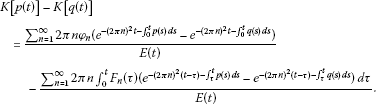Using the estimateswe obtain

$\underset{0\le t\le T}{max}|K\left[p\left(t\right)\right]-K\left[q\left(t\right)\right]|\le \alpha \underset{0\le t\le T}{max}|p\left(t\right)-q\left(t\right)|.$

Let $\alpha \in \left(0,1\right)$ be an arbitrary fixed number. Fix a number ${T}_{0}$, $0<{T}_{0}\le T$, such that

$\frac{{C}_{3}+{C}_{4}}{{C}_{0}}{T}_{0}\le \alpha ,$

where

${C}_{0}=\underset{t\in \left[0,T\right]}{min}E\left(t\right),\phantom{\rule{2em}{0ex}}{C}_{3}=\sum _{n=1}^{\mathrm{\infty }}2\pi n{\phi }_{n},\phantom{\rule{2em}{0ex}}{C}_{4}=T\underset{t\in \left[0,T\right]}{max}\left(\sum _{n=1}^{\mathrm{\infty }}2\pi n{F}_{n}\left(t\right)\right).$

Then, from equality (10), we obtain

${\parallel p-q\parallel }_{C\left[0,{T}_{0}\right]}\le \alpha {\parallel p-q\parallel }_{C\left[0,{T}_{0}\right]},$

which implies that $p=q$. By substituting $p=q$ in (9), we have $u=v$. □

## 3 Continuous dependence of $\left(p,u\right)$ upon the data

Theorem 3 Under assumptions (A1)-(A3), the solution $\left(p,u\right)$ of the problem (1)-(4) depends continuously upon the data for small T.

The proof of the theorem is verified by analogy to .

## 4 Numerical method

We use the finite difference method with a predictor-corrector type approach that is suggested in . Apply this method to the problem (1)-(4).

We subdivide the intervals $\left[0,1\right]$ and $\left[0,T\right]$ into ${N}_{x}$ and ${N}_{t}$ subintervals of equal lengths $h=\frac{1}{{N}_{x}}$ and $\tau =\frac{T}{{N}_{t}}$, respectively. Then we add two lines $x=0$ and $x=\left({N}_{x}+1\right)h$ to generate the fictitious points needed for dealing with the boundary conditions. We choose the Crank-Nicolson scheme, which is absolutely stable and has a second-order accuracy in both h and τ.  The Crank-Nicolson scheme for (1)-(4) is as follows: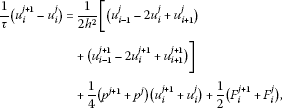(11)(12)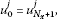(13)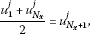(14)

where $1\le i\le {N}_{x}$ and $0\le j\le {N}_{t}$ are the indices for the spatial and time steps respectively, ${u}_{i}^{j}=u\left({x}_{i},{t}_{j}\right)$, ${\varphi }_{i}=\phi \left({x}_{i}\right)$, ${F}_{i}^{j}=F\left({x}_{i},{t}_{j}\right)$, ${x}_{i}=ih$, ${t}_{j}=j\tau$. At the $t=0$ level, adjustment should be made according to the initial condition and the compatibility requirements.

Equations (11)-(14) form an ${N}_{x}×{N}_{x}$ linear system of equations

$A{U}^{j+1}=b,$
(15)

whereNow, let us construct the predicting-correcting mechanism. First, integrating equation (1) with respect to x from 0 to 1 and using (3) and (4), we obtain

$p\left(t\right)=\frac{-{E}^{\mathrm{\prime }}\left(t\right)+{\int }_{0}^{1}xF\left(x,t\right)\phantom{\rule{0.2em}{0ex}}dx+{u}_{x}\left(1,t\right)}{E\left(t\right)}.$
(16)

The finite difference approximation of (16) is

${p}^{j}=\frac{-\left(\left({E}^{j+1}-{E}^{j}\right)/\tau \right)+{\left(Fin\right)}^{j}+\left({u}_{{N}_{x}+1}^{j}-{u}_{{N}_{x}}^{j}\right)/h}{{E}^{j}},$

where ${E}^{j}=E\left({t}_{j}\right)$, ${\left(Fin\right)}^{j}={\int }_{0}^{1}xF\left(x,{t}_{j}\right)\phantom{\rule{0.2em}{0ex}}dx$, $j=0,1,\dots ,{N}_{t}$.

For $j=0$,

${p}^{0}=\frac{-\left(\left({E}^{1}-{E}^{0}\right)/\tau \right)+{\left(Fin\right)}^{0}+\left({\varphi }_{{N}_{x}+1}-{\varphi }_{{N}_{x}}\right)/h}{{E}^{j}},$

and the values of ${\varphi }_{i}$ allow us to start our computation. We denote the values of ${p}^{j}$, ${u}_{i}^{j}$ at the s th iteration step ${p}^{j\left(s\right)}$, ${u}_{i}^{j\left(s\right)}$, respectively. In numerical computation, since the time step is very small, we can take ${p}^{j+1\left(0\right)}={p}^{j}$, ${u}_{i}^{j+1\left(0\right)}={u}_{i}^{j}$, $j=0,1,2,\dots ,{N}_{t}$, $i=1,2,\dots ,{N}_{x}$. At each $\left(s+1\right)$th iteration step, we first determine ${p}^{j+1\left(s+1\right)}$ from the formula

${p}^{j+1\left(s+1\right)}=\frac{-\left(\left({E}^{j+2}-{E}^{j}+1\right)/\tau \right)+{\left(Fin\right)}^{j+1}+\left({u}_{{N}_{x}+1}^{j+1\left(s\right)}-{u}_{{N}_{x}}^{j+1\left(s\right)}\right)/h}{{E}^{j}}.$

Then from (11)-(14) we obtain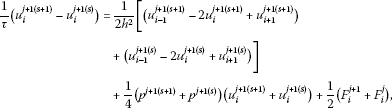(17)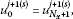(18)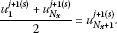(19)

The system of equations (17)-(19) can be solved by the Gauss elimination method, and ${u}_{i}^{j+1\left(s+1\right)}$ is determined. If the difference of values between two iterations reaches the prescribed tolerance, the iteration is stopped, and we accept the corresponding values ${p}^{j+1\left(s+1\right)}$, ${u}_{i}^{j+1\left(s+1\right)}$ ($i=1,2,\dots ,{N}_{x}$) as ${p}^{j+1}$, ${u}_{i}^{j+1}$ ($i=1,2,\dots ,{N}_{x}$), on the $\left(j+1\right)$th time step, respectively. By virtue of this iteration, we can move from level j to level $j+1$.

## 5 Numerical example and discussions

In this section, we present examples to illustrate the efficiency of the numerical method described in the previous section.

Example Consider the inverse problem (1)-(4) with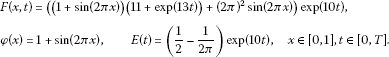It is easy to check that the analytical solution of this problem is

$\left\{p\left(t\right),u\left(x,t\right)\right\}=\left\{1+exp\left(13t\right),\left(1+sin\left(2\pi x\right)\right)exp\left(10t\right)\right\}.$
(20)

Let us apply the scheme which was explained in the previous section for the step sizes $h=0.01$, $\tau =\frac{h}{8}$.

In the case when $T=1/2$, the comparisons between the analytical solution (19) and the numerical finite difference solution are shown in Figures 1 and 2.

## 6 Conclusions

The inverse problem regarding the simultaneous identification of the time-dependent coefficient of heat capacity together with the temperature distribution in a one-dimensional heat equation with periodic boundary and integral overdetermination conditions has been considered. This inverse problem has been investigated from both theoretical and numerical points of view. In the theoretical part of the article, the conditions for the existence, uniqueness and continuous dependence upon the data of the problem have been established. In the numerical part, a numerical example using the Crank-Nicolson finite-difference scheme combined with the iteration method is presented.

## References

1. Cannon J, Lin Y, Wang S: Determination of a control parameter in a parabolic partial differential equation. J. Aust. Math. Soc. Ser. B, Appl. Math 1991, 33: 149–163. 10.1017/S0334270000006962

2. Cannon J, Lin Y, Wang S: Determination of source parameter in a parabolic equations. Meccanica 1992, 27: 85–94. 10.1007/BF00420586

3. Fatullayev A, Gasilov N, Yusubov I: Simultaneous determination of unknown coefficients in a parabolic equation. Appl. Anal. 2008, 87: 1167–1177. 10.1080/00036810802140616

4. Ivanchov M, Pabyrivska N: Simultaneous determination of two coefficients of a parabolic equation in the case of nonlocal and integral conditions. Ukr. Math. J. 2001, 53: 674–684. 10.1023/A:1012570031242

5. Ismailov M, Kanca F: An inverse coefficient problem for a parabolic equation in the case of nonlocal boundary and overdetermination conditions. Math. Methods Appl. Sci. 2011, 34: 692–702. 10.1002/mma.1396

6. Kanca F, Ismailov M: Inverse problem of finding the time-dependent coefficient of heat equation from integral overdetermination condition data. Inverse Probl. Sci. Eng. 2012, 20: 463–476. 10.1080/17415977.2011.629093

7. Ionkin N: Solution of a boundary-value problem in heat conduction with a nonclassical boundary condition. Differ. Equ. 1977, 13: 204–211.

8. Vigak V: Construction of a solution of the heat-conduction problem with integral conditions. Dokl. Akad. Nauk Ukr. SSR 1994, 8: 57–60.

9. Ivanchov N: Boundary value problems for a parabolic equation with integral conditions. Differ. Equ. 2004, 40: 591–609.

10. Choi J: Inverse problem for a parabolic equation with space-periodic boundary conditions by a Carleman estimate. J. Inverse Ill-Posed Probl. 2003, 11: 111–135. 10.1515/156939403766493519

11. Ashyralyev A, Erdogan A: On the numerical solution of a parabolic inverse problem with the Dirichlet condition. Int. J. Math. Comput. 2011, 11: 73–81.

12. Sakinc I: Numerical solution of the quasilinear parabolic problem with periodic boundary condition. Hacet. J. Math. Stat. 2010, 39: 183–189.

13. Samarskii AA: The Theory of Difference Schemes. Dekker, New York; 2001.

## Acknowledgements

Dedicated to Professor Hari M Srivastava.

## Author information

Authors

### Corresponding author

Correspondence to Fatma Kanca.

### Competing interests

The author declares that they have no competing interests.

## Authors’ original submitted files for images

Below are the links to the authors’ original submitted files for images.

## Rights and permissions

Reprints and Permissions

Kanca, F. The inverse problem of the heat equation with periodic boundary and integral overdetermination conditions. J Inequal Appl 2013, 108 (2013). https://doi.org/10.1186/1029-242X-2013-108

• Accepted:

• Published:

• DOI: https://doi.org/10.1186/1029-242X-2013-108

### Keywords

• Inverse Problem
• Finite Difference Method
• Continuous Dependence
• Fourier Method
• Parabolic Differential Equation